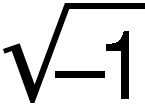Definition: pi from Philip's Encyclopedia

(π) Symbol used for the ratio of the circumference of a circle to its diameter. It is an irrational number, and an approximation to five decimal places is 3.14159. The ratio 22/7 is often used as a rougher approximation.

Summary Article: pi
From The Columbia Encyclopedia

in mathematics, the ratio of the circumference of a circle to its diameter. The symbol for pi is π. The ratio is the same for all circles and is approximately 3.1416. It is of great importance in mathematics not only in the measurement of the circle but also in more advanced mathematics in connection with such topics as continued fractions, logarithms of imaginary numbers, and periodic functions. Throughout the ages progressively more accurate values have been found for π; an early value was the Greek approximation 3 1/7, found by considering the circle as the limit of a series of regular polygons with an increasing number of sides inscribed in the circle. About the mid-19th cent. its value was figured to 707 decimal places and by the mid-20th cent. an electronic computer had calculated it to 100,000 digits. Although it has now been calculated to some 2.6 trillion digits, the exact value of π cannot be computed. It was shown by the German mathematician Johann Lambert in 1770 that π is irrational and by Ferdinand Lindemann in 1882 that π is transcendental; i.e., cannot be the root of any algebraic equation with rational coefficients. The important connection between π and e, the base of natural logarithms, was found by Leonhard Euler in the famous formula e=−1, where i=.The Columbia Encyclopedia, © Columbia University Press 2018

### Related Articles

##### Full text Article pi
The Penguin Dictionary of Mathematics

Symbol: π. The ratio of the length of the circumference of a circle to its diameter. The symbol π was first used in this sense by the Welsh...

##### Full text Article III.70 π
The Princeton Companion to Mathematics

What makes one number more fundamental and important, mathematically speaking, than another? Why, for instance, would almost everybody agree that...

##### Full text Article pi
Britannica Concise Encyclopedia

In mathematics, the ratio of the circumference of a circle to its diameter. An irrational number (see also transcendental number), it has an approx

See more from Credo